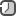### Date workout in Java

Java's `java.util.Calendar` class is used to do date and time arithmetic. Whenever you have something slightly more advanced than just representing a date and time, this is the class to use.

Java only comes with a `Gregorian` calendar implementation, the `java.util.GregorianCalendar` class. Here is how you instantiate a `GregorianCalendar`:

```Calendar calendar = new GregorianCalendar();
```

The `Calendar` class has a couple of methods you can use to access the year, month, day, hour, minutes, seconds, milliseconds and time zone of a given date. Here are a few examples showing how that is done:

```Calendar calendar = new GregorianCalendar();

int year = calendar.get(Calendar.YEAR);
int month = calendar.get(Calendar.MONTH);
int dayOfMonth = calendar.get(Calendar.DAY_OF_MONTH); // Jan = 0, not 1
int dayOfWeek = calendar.get(Calendar.DAY_OF_WEEK);
int weekOfYear = calendar.get(Calendar.WEEK_OF_YEAR);
int weekOfMonth= calendar.get(Calendar.WEEK_OF_MONTH);

int hour = calendar.get(Calendar.HOUR);        // 12 hour clock
int hourOfDay = calendar.get(Calendar.HOUR_OF_DAY); // 24 hour clock
int minute = calendar.get(Calendar.MINUTE);
int second = calendar.get(Calendar.SECOND);
int millisecond = calendar.get(Calendar.MILLISECOND);
```

The `MONTH` field of the `Calendar` class does not go from 1 to 12 like they do when we write dates otherwise. Instead the months run from 0 to 11, where 0 is January and 11 is December.

The day of week runs from 1 to 7 as you might expect, but sunday, not monday is the first day of the week. That means that 1 = sunday, 2 = monday, ..., 7 = saturday.

How to add/subtract year, months, days, hour, minutes

Java’s `Calendar` class provides a set of methods for manipulation of temporal information. In addition to fetch the system’s current date and time, it also enables functionality for date and time arithmetic.

```Calendar cal = new GregorianCalendar(2017, Calendar.JUNE, 2);

// add 4 months and 5 days

// add 3 hours and 34 minute

// subtract 4 months,5 days,12 hours and 24 minutes
```

How to get current timestamp

```//Date object
Date date= new Date();
//getTime() returns current time in milliseconds
long timeMilli1 = date.getTime();

Calendar calendar = Calendar.getInstance();
long timeMilli2 = calendar.getTimeInMillis();

long timeMili3 = System.currentTimeMillis();
```

Date comparison

```// Initialization

Calendar startDate = Calendar.getInstance();
startDate.set(2013, 11, 25);

Calendar endDate = Calendar.getInstance();
endDate.set(2013, 11, 27);

// Check if startDate is equal to endDate
startDate.equals(endDate)

// Check if startDate is less than endDate
startDate.before(endDate)

// check if startDate is greater than endDate
startDate.after(endDate)
```

Difference between two dates

Difference between two dates using `TimeUnit` and `Calendar`.

```Calendar calStart = Calendar.getInstance();
calStart.set(2013, 11, 25);

Calendar calEnd = Calendar.getInstance();
calEnd.set(2013, 11, 27);

Date dateStart = calStart.getTime();
Date dateEnd = calEnd.getTime();

long timeDiff = Math.abs(dateEnd.getTime() - dateStart.getTime());
String diff = String.format("%d hour(s) %d min(s)", TimeUnit.MILLISECONDS.toHours(timeDiff),
TimeUnit.MILLISECONDS.toMinutes(timeDiff) - TimeUnit.HOURS.toMinutes(TimeUnit.MILLISECONDS.toHours(timeDiff)));
System.out.println(diff);
```

Difference between two dates using `Calendar`.

```// import java.util.Calendar;

Calendar cal1 = Calendar.getInstance();
Calendar cal2 = Calendar.getInstance();

// Set the date for both of the calendar instance
cal1.set(2016, Calendar.DECEMBER, 30);
cal2.set(2017, Calendar.MAY, 3);

// Get the represented date in milliseconds
long millis1 = cal1.getTimeInMillis();
long millis2 = cal2.getTimeInMillis();

// Calculate difference in milliseconds
long diff = millis2 - millis1;

// Calculate difference in seconds
long diffSeconds = diff / 1000;

// Calculate difference in minutes
long diffMinutes = diff / (60 * 1000);

// Calculate difference in hours
long diffHours = diff / (60 * 60 * 1000);

// Calculate difference in days
long diffDays = diff / (24 * 60 * 60 * 1000);

System.out.println("In milliseconds: " + diff + " milliseconds.");
System.out.println("In seconds: " + diffSeconds + " seconds.");
System.out.println("In minutes: " + diffMinutes + " minutes.");
System.out.println("In hours: " + diffHours + " hours.");
System.out.println("In days: " + diffDays + " days.");
```

Difference between two dates using `LocalDate` (JDK 8), `Month` and `Period`.

```//import java.time.LocalDate;
//import java.time.Month;
//import java.time.Period;

LocalDate dateFrom = LocalDate.of(2016, Month.JULY, 12);
LocalDate dateTo = LocalDate.of(2017, Month.AUGUST, 22);

Period intervalPeriod = Period.between(dateFrom, dateTo);

System.out.println("Difference of days: " + intervalPeriod.getDays());
System.out.println("Difference of months: " + intervalPeriod.getMonths());
System.out.println("Difference of years: " + intervalPeriod.getYears());
```

Difference between two dates using `LocalDate` (JDK 8), `Month` and `ChronoUnit`.

```//import java.time.LocalDate;
//import java.time.Month;
//import java.time.temporal.ChronoUnit;

LocalDate dateFrom = LocalDate.of(2016, Month.JULY, 12);
LocalDate dateTo = LocalDate.of(2017, Month.AUGUST, 22);

long intervalYears = ChronoUnit.YEARS.between(dateFrom, dateTo);
System.out.println("Total number of years between dates: " + intervalYears);

long intervalMonths = ChronoUnit.MONTHS.between(dateFrom, dateTo);
System.out.println("Total number of months between dates: " + intervalMonths);

long intervalDays = ChronoUnit.DAYS.between(dateFrom, dateTo);
System.out.println("Total number of days between dates:" + intervalDays);
```

Difference between two dates using `LocalDate` from Joda-Time library.

```LocalDate date1 = new LocalDate(2015, 7, 30);
LocalDate date2 = new LocalDate(2017, 5, 19);

int monthsBetween = Months.monthsBetween(date1, date2).getMonths();
int yearsBetween = Years.yearsBetween(date1, date2).getYears();
```

Difference between two dates with Joda-time library.

```import java.text.ParseException;
import java.text.SimpleDateFormat;
import java.util.Date;

import org.joda.time.Interval;
import org.joda.time.Period;

public class JodaTest {
public static void main(String[] args) {
SimpleDateFormat sdf = new SimpleDateFormat("dd/M/yyyy HH:mm:ss");
try {
Date startDate = sdf.parse("25/11/2013 21:45:05");
Date endDate = sdf.parse("27/11/2013 23:03:12");

Interval interval = new Interval(startDate.getTime(), endDate.getTime());
Period period = interval.toPeriod();

System.out.printf("%d days, %d hours, %d minutes, %d seconds%n",
period.getDays(), period.getHours(), period.getMinutes(), period.getSeconds());

System.out.println(period.getMonths());
} catch (ParseException e) {
e.printStackTrace();
}
}
}
```

Run with this command

```javac -cp joda-time-2.3.jar JodaTest.java && java -cp .:joda-time-2.3.jar JodaTest
```

Date formation

The `DateFormat` class provides APIs for formatting time and date in a custom format.

```String frmt = "MM/dd/yy HH:mm";
Date today = new Date();
DateFormat.format(frmt, today);
```

In this snippet we will use `Calendar` and `SimpleDateFormate` class to format current date.

```SimpleDateFormat sdf = new SimpleDateFormat("dd/MM/yyyy");
Calendar c = Calendar.getInstance();
String date1 = sdf.format(c.getTime());
String date2 = sdf.format(new Date());
```

We can use `Calendar` class to get current date in another way.

```Calendar c = Calendar.getInstance();
int day = c.get(Calendar.DAY_OF_MONTH);
int month = c.get(Calendar.MONTH);
int year = c.get(Calendar.YEAR);
String date = day + "/" + (month+1) + "/" + year;
```

How to convert String to Date

You can parse a `String` into a `java.util.Date` instance using the `parse(`) method of the `SimpleDateFormat` instance. Here is an example:

```Date date = null;
DateFormat df = new SimpleDateFormat("dd-MM-yyyy");

try {
date = df.parse("02-07-2017");
} catch (Exception e){
System.out.println(e);
}
```